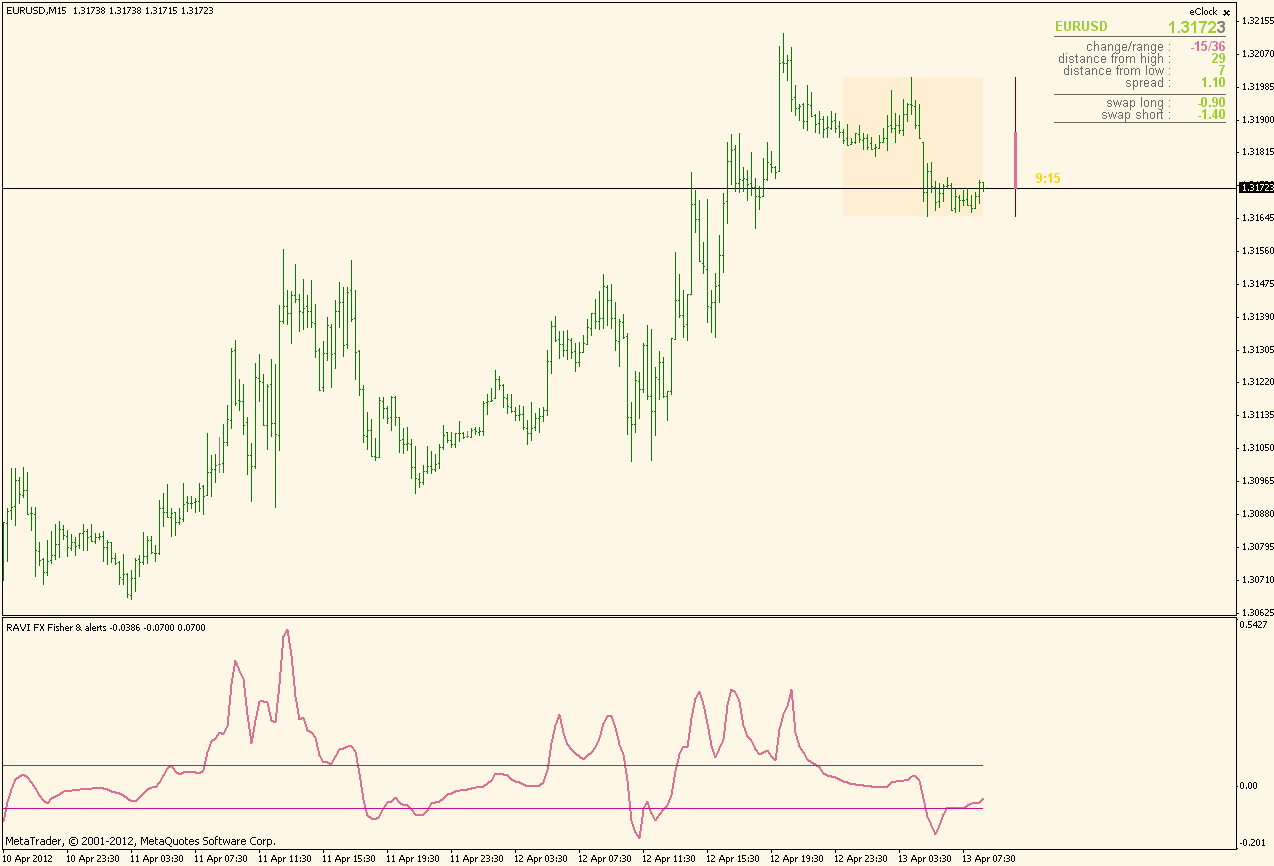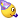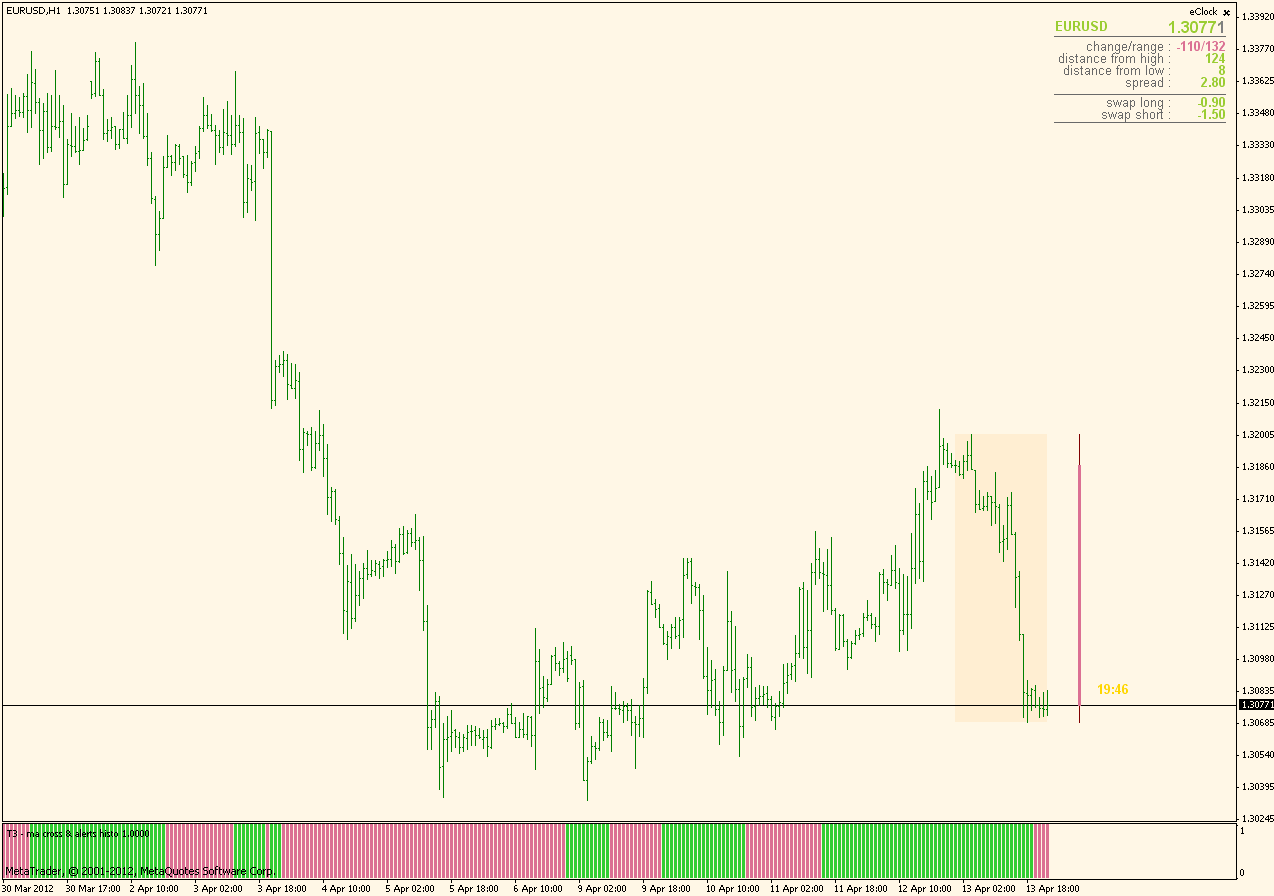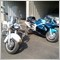# Indicators with alerts/signal - page 3699493

wolfsch:
Hi Mrtools

wolfsch

btw: I still dont know how to post an indi or chart to the thread. It would be great to get an advice!

Thanks

Oops sorry i missed that, what i did now is reply with quote then scroll down until you see manage attachments click on that, when the box comes up click on add files, then after box gets smaller click on basic uploader, then browse, click on file you want to upload, then upload, when they upload they are in your attachment area you can choose to insert them inline or not, i just uncheck the little box in the attachments, to not insert them inline, but thats just my preference.480

mrtools

mrtools:
Oops sorry i missed that, what i did now is reply with quote then scroll down until you see manage attachments click on that, when the box comes up click on add files, then after box gets smaller click on basic uploader, then browse, click on file you want to upload, then upload, when they upload they are in your attachment area you can choose to insert them inline or not, i just uncheck the little box in the attachments, to not insert them inline, but thats just my preference.

Hi

thanks this is just a try!

Files:10

mrtools:
Hi Kidrock,

Wish i could help you but the decompiling really makes the code hard to figure out if we had the true source making alerts for it could be done.

ps) did find this one from nen, looks pretty good and has alerts.

Mrtools thx for the reply, i didnt know that decompiling an ex4 make the code harder to figured out, why is that?

so there's nothing i cant do? i also have nen indi but this one is better for me10

Here you goIt will alert when "trigger" value is crossed (so you can configure it from parameters) and it will also alert you when it reverts from upper or lower zone. Also, a bars limit is removed (it calculates on the whole history now)Thanks mladen, that is exactly what I needed10

mt4-stochasticrsi-oscillator.mq4

Thanks.

Files:9493

kidrock:
Mrtools thx for the reply, i didnt know that decompiling an ex4 make the code harder to figured out, why is that? so there's nothing i cant do? i also have nen indi but this one is better for meMladen explained it very well somewhere which i can't find, but check out some of the decompiled code

int start() {

int lia_0;

double lda_4;

double ld_28;

double ld_36;

if (g_bars_108 == Bars) return (0);

delete_obj();

g_bars_108 = Bars;

int li_unused_12 = 0;

int li_16 = 4;

int li_20 = gi_80;

int li_24 = -1;

while (li_20 < g_bars_100) {

while (li_20 = 0) {

if (gda_96[li_20] != 0.0) {

lia_0[li_16] = li_20;

lda_4[li_16] = gda_96[li_20];

li_16--;

}

li_20++;

}

ld_28 = lda_4 * (lda_4 - lda_4);

ld_36 = lda_4 * (lda_4 - lda_4);

if ((ld_28 - lda_4) * (ld_36 - lda_4) <= 0.0 && (lda_4 - lda_4) * (lda_4 - lda_4) ) {

if (lda_4 < lda_4 && lda_4 < lda_4 && (lda_4 - lda_4) * (lda_4 - lda_4) ) {

li_24 = li_20 - 1;

} else {

if (lda_4 > lda_4 && lda_4 > lda_4 && (lda_4 - lda_4) * (lda_4 - lda_4) ) {

li_24 = li_20 - 1;

}

}

}

for (int li_44 = 4; li_44 > 0; li_44--) {

lia_0[li_44] = lia_0[li_44 - 1];

lda_4[li_44] = lda_4[li_44 - 1];

}

li_16 = 0;

Sleep(20);

if (first_one && li_24 != -1) break;

}[/PHP]

Probably not a good example but this is regular code

[PHP]int start()

{

int counted_bars=IndicatorCounted();

int i,limit;

if(counted_bars<0) return(-1);

if(counted_bars>0) counted_bars--;

limit = MathMin(Bars-counted_bars,Bars-1);

//

//

//

//

//

for (i=limit; i>=0; i--) temp = MathMax(High,Close) - MathMin(Low,Close);

for (i=limit; i>=0; i--)

{

atr = iMAOnArray(temp,Bars,AtrPeriod,0,AtrMa_Mode,i);

}

for (i=limit; i>=0; i--) atrma = iMAOnArray(atr, Bars,MaPeriod,0,Ma_Mode,i);

return(0);

}

Also for whatever reason the original author didn't want the code seen, something i think we should respect.10

mrtools:
Mladen explained it very well somewhere which i can't find, but check out some of the decompiled code
int start() {

int lia_0;

double lda_4;

double ld_28;

double ld_36;

if (g_bars_108 == Bars) return (0);

delete_obj();

g_bars_108 = Bars;

int li_unused_12 = 0;

int li_16 = 4;

int li_20 = gi_80;

int li_24 = -1;

while (li_20 < g_bars_100) {

while (li_20 = 0) {

if (gda_96[li_20] != 0.0) {

lia_0[li_16] = li_20;

lda_4[li_16] = gda_96[li_20];

li_16--;

}

li_20++;

}

ld_28 = lda_4 * (lda_4 - lda_4);

ld_36 = lda_4 * (lda_4 - lda_4);

if ((ld_28 - lda_4) * (ld_36 - lda_4) <= 0.0 && (lda_4 - lda_4) * (lda_4 - lda_4) ) {

if (lda_4 < lda_4 && lda_4 < lda_4 && (lda_4 - lda_4) * (lda_4 - lda_4) ) {

li_24 = li_20 - 1;

} else {

if (lda_4 > lda_4 && lda_4 > lda_4 && (lda_4 - lda_4) * (lda_4 - lda_4) ) {

li_24 = li_20 - 1;

}

}

}

for (int li_44 = 4; li_44 > 0; li_44--) {

lia_0[li_44] = lia_0[li_44 - 1];

lda_4[li_44] = lda_4[li_44 - 1];

}

li_16 = 0;

Sleep(20);

if (first_one && li_24 != -1) break;

}[/PHP]

Probably not a good example but this is regular code

[PHP]int start()

{

int counted_bars=IndicatorCounted();

int i,limit;

if(counted_bars<0) return(-1);

if(counted_bars>0) counted_bars--;

limit = MathMin(Bars-counted_bars,Bars-1);

//

//

//

//

//

for (i=limit; i>=0; i--) temp = MathMax(High,Close) - MathMin(Low,Close);

for (i=limit; i>=0; i--)

{

atr = iMAOnArray(temp,Bars,AtrPeriod,0,AtrMa_Mode,i);

}

for (i=limit; i>=0; i--) atrma = iMAOnArray(atr, Bars,MaPeriod,0,Ma_Mode,i);

return(0);

}
Also for whatever reason the original author didn't want the code seen, something i think we should respect.

I see, they are quite different... thanks anyway153865

T3 - ma crosses with alerts ...

This is a T3 - ma crosses indicator from this post : https://www.mql5.com/en/forum/173058/page15 with alerts added

Files:153865

T3 ma crosses - histgram version ...Files:256